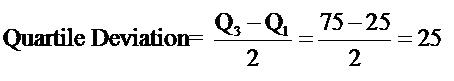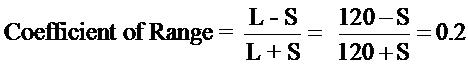Courses

# Test: Measures Of Dispersion

## 10 Questions MCQ Test Economics Class 11 | Test: Measures Of Dispersion

Description
This mock test of Test: Measures Of Dispersion for Commerce helps you for every Commerce entrance exam. This contains 10 Multiple Choice Questions for Commerce Test: Measures Of Dispersion (mcq) to study with solutions a complete question bank. The solved questions answers in this Test: Measures Of Dispersion quiz give you a good mix of easy questions and tough questions. Commerce students definitely take this Test: Measures Of Dispersion exercise for a better result in the exam. You can find other Test: Measures Of Dispersion extra questions, long questions & short questions for Commerce on EduRev as well by searching above.
QUESTION: 1

### Quartile deviation with Q1 = 25 and Q3 = 75 is equal to

Solution:QUESTION: 2

### Measure of dispersion considering only extreme values is called

Solution:

Range is the difference between the largest value and the smallest value of a series.
Range = L- S
Here,  L= Largest value, S= Smallest value.
No other values of the series are taken into consideration in this case.

QUESTION: 3

### The following is a graphic method of measuring dispersion

Solution:

The departure of the Lorenz curve from the line of equal distribution would indicate the extent of dispersion.

QUESTION: 4

Lower value of quartile deviation implies

Solution:

Half of the Inter-Quartile Range is called Quartile Deviation. Lower the value of the quartile deviation, lower the dispersion.

QUESTION: 5

To measure income inequality we can use

Solution:

A measure of dispersion can tell us about income inequalities.

QUESTION: 6

Coefficient of Quartile deviation, with Q1 at 25 and Q3 at 125, equals

Solution:

The coefficient of quartile deviation (sometimes called the quartile coefficient of dispersion) allows you to compare dispersion for two or more sets of data. The formula is:
Q3-Q1
Q3+Q1
100/150= 0.66

QUESTION: 7

The relative measures of dispersion are expressed in

Solution:

The relative measures of dispersion are expressed in percentage. These are used to compare two or more series which are expressed in different units.

QUESTION: 8

The smallest value of a series, with largest value at 120 and coefficient of range at 0.2, equals

Solution:S = 80

QUESTION: 9

The graphical method of studying dispersion is known as

Solution:

The graphical method of studying dispersion is known as Lorenz curve method. It is named after Dr. Max. O. Lorenz who used it for the first time to measure the distribution of income and wealth.

QUESTION: 10

The best measure of dispersion is

Solution:

Standard deviation is the best and widely used measure of dispersion. The properties of standard deviation are:
(i) Standard deviation is rigidly defined.
(ii) It requires harder calculations.
(iii) It depends on all the values of the variable.
(iv) It is based on deviations from the arithmetic mean.
(v) It is capable of further statistical treatment.